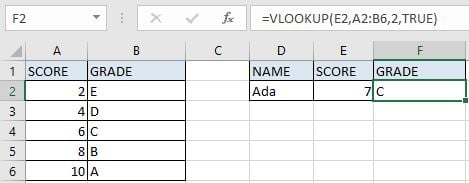# Basic Grade Calculation by VLOOKUP Function – Approximate Match

In Excel, except combination INDEX+MATCH, we can also apply other functions to search data, for example VLOOKUP function. Like MATCH function, VLOOKUP function is one of Excel lookup & reference functions that can perform approximate match or exact match by setting lookup matching modes.

Today, we will show you the way to calculate “Grade” by VLOOKUP function. In this instance, VLOOKUP will perform an approximate match when scanning values from the given table. If you meet similar scenarios in your daily work, you can directly use the formula with the help of VLOOKUP function we created in this case to deal with your problem.

## EXAMPLEIn this example, we want to get proper grade in F2 based on the input score in E2. The corresponding relationship between score and grade you can refer to the left table. As the table only provides the starting value of score for each grade, so we can look up the input score from this table “Score” column by performing an approximate match, then returns grade from “Grade” for the corresponding staring value properly.

In this article, to approach our goal, we will apply VLOOKUP function to create formula.

## ANALYSIS

To return proper grade based on the input score:

1. There is only one condition to determine which grade is returned: score.

2. In this example, our input score is “7” is not listed directly in “Score” column, only the starting values of each score range are listed, so we can find the starting value of our input score “7” depends on performing an approximate match.

3. Through scanning each starting value from “Score” column, we can confirm “7” belongs to which score range at last by some rules; then based on the starting value of this range, we can return corresponding grade from adjacent “Grade” column properly.

In Excel, except the good partners INDEX and MATCH, we can also select some other functions to look up values. VLOOKUP is one of the most frequently used functions in Excel that can perform searching data properly, it also can perform approximate match or exact match by setting match mode.

Above all, we create below formula to solve this problem:

=VLOOKUP(E2,A2:B6,2,TRUE)

## FORMULA

Input formula =VLOOKUP(E2,A2:B6,2,TRUE) into F2, press ENTER, verify that grade “C” is returned properly.Values in “Score” column are the starting values for each score range. Refer to normal approximate match rules, grade “C” is the corresponding grade for score which is greater than or equal to 6, grade “B” is for score which is greater than or equal to 8, so “C” is returned for score 7. More details please see below Explanation part.

## FUNCTION INTRODUCTION

a. VLOOKUP function looks up the input value from the first column of the given table, and returns the value we want from a particular position (row number depends on the row of lookup value, column number depends on the input argument col_index_num).

Syntax:

`=VLOOKUP(lookup_value, table_array, col_index_num,[range_lookup])`

For argument “range_lookup”, there are two matching modes “True” and “False”.

• The default mode is “True”, so if this optional argument is omitted, this value is set to “True” automatically.
• If we set “range_lookup” to “True”, VLOOKUP function performs an approximate match.
• If we set “range_lookup” to “False”, VLOOKUP function looks up data restrictively by performing an exact match. If look up value is not found in table, formula returns error “#N/A”.

## EXPLANATION

`=VLOOKUP(E2,A2:B6,2,TRUE)`

lookup_value is input into D2 (“7”)

table_array is “A2:B6”,

col_index_num is 2. Because grades are saved in the second column of this table.

range_lookup is set to TRUE. VLOOKUP function performs an approximate match.

a. In this formula, the look up value is “7”, VLOOKUP scans this value from the first column of the given table, in this case it is the range “A2:A6”. It continues scanning until it meets the value that is greater than the lookup value. In this case, VLOOKUP function will stop scanning when meeting “8” and returns to previous row of “6”.

b. The given table_array is range “A2:B6”, this table contains the lookup value in its first column and also contains the grade we want to return by the formula, so we input “A2:B6” as table_array, just input a single column doesn’t work.

c. As the grades are listed in the second column of A2:B6, so the col_index_num is 2. So, VLOOKUP function retrieves data at the intersection of the second column and the row of score “6”.

## EXPAND

a. In Excel, INDEX function and MATCH function are often used together for returning value or cell reference/range reference from a particular position. So, we can also create below formula contains INDEX and MATCH to help us solve the problem:

=INDEX(B2:B6,MATCH(E2,A2:A6,1))

a. When performing an approximate match of VLOOKUP function, the lookup values must be sorted in ascending order in precondition. If values in the first column are in disorder, VLOOKUP function will stop scanning if it meets the first value that is greater than the lookup value, thus the returned value is improper.

### Related Functions

• Excel INDEX function
The Excel INDEX function returns a value from a table based on the index (row number and column number)The INDEX function is a build-in function in Microsoft Excel and it is categorized as a Lookup and Reference Function.The syntax of the INDEX function is as below:= INDEX (array, row_num,[column_num])…
• Excel MATCH  function
The Excel MATCH function search a value in an array and returns the position of that item.The MATCH function is a build-in function in Microsoft Excel and it is categorized as a Lookup and Reference Function.The syntax of the MATCH function is as below:= MATCH  (lookup_value, lookup_array, [match_type])….
• Excel VLOOKUP function
The Excel VLOOKUP function lookup a value in the first column of the table and return the value in the same row based on index_num position.The syntax of the VLOOKUP function is as below:= VLOOKUP (lookup_value, table_array, column_index_num,[range_lookup])….